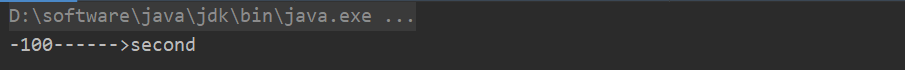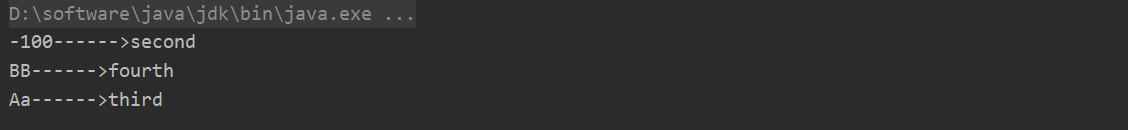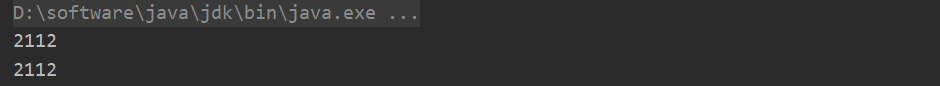# Java入门系列之集合Hashtable源码分析

## Hashtable源码分析

 public static void main(String[] args) {

Hashtable hashtable = new Hashtable();
hashtable.put(-100, "first");
}

public class Hashtable<K,V>
extends Dictionary<K,V>
implements Map<K,V>, Cloneable, java.io.Serializable {

//存储键值对数据
private transient Entry<?,?>[] table;

//存储数据大小
private transient int count;

private int threshold;

//负载因子: 从时间和空间成本折衷考虑默认为0.75。因为较高的值虽然会减少空间开销，但是增加查找元素的时间成本

//指定容量和负载因子构造函数
public Hashtable(int initialCapacity, float loadFactor) {
if (initialCapacity < 0)
throw new IllegalArgumentException("Illegal Capacity: "+
initialCapacity);

if (initialCapacity==0)
initialCapacity = 1;

table = new Entry<?,?>[initialCapacity];

//默认阈值为8
threshold = (int)Math.min(initialCapacity * loadFactor, MAX_ARRAY_SIZE + 1);
}

//指定容量构造函数
public Hashtable(int initialCapacity) {
this(initialCapacity, 0.75f);
}

//默认无参构造函数（初始化容量为11，负载因子为0.75f)
public Hashtable() {
this(11, 0.75f);
}

private static class Entry<K,V> implements Map.Entry<K,V> {
final int hash;
final K key;
V value;
Entry<K,V> next;

protected Entry(int hash, K key, V value, Entry<K,V> next) {
this.hash = hash;
this.key =  key;
this.value = value;
this.next = next;
}
}
}

Hashtable内部通过Entry数组存储数据，通过Entry结构可看出采用链地址法解决哈希冲突，当初始化Hashtable未指定容量和负载因子时，默认初始化容量为11，负载因子为0.75，阈值为8，若容量小于0则抛出异常，若容量等于0则容量为1且阈值为0，否则阈值以指定容量*0.75计算或者以指定容量*指定负载因子计算为准。

public synchronized V put(K key, V value) {
if (value == null) {
throw new NullPointerException();
}

Entry<?,?> tab[] = table;

int hash = key.hashCode();

int index = (hash & 0x7FFFFFFF) % tab.length;

Entry<K,V> entry = (Entry<K,V>)tab[index];

for(; entry != null ; entry = entry.next) {
if ((entry.hash == hash) && entry.key.equals(key)) {
V old = entry.value;
entry.value = value;
return old;
}
}

return null;
}    

 int index = (hash & 0x7FFFFFFF) % tab.length;

       for(; entry != null ; entry = entry.next) {
if ((entry.hash == hash) && entry.key.equals(key)) {
V old = entry.value;
entry.value = value;
return old;
}
}

public static void main(String[] args) {

Hashtable hashtable = new Hashtable();

hashtable.put(-100, "first");

hashtable.put(-100, "second");
}

        Enumeration keys = hashtable.keys();

while (keys.hasMoreElements()) {

Object key =  keys.nextElement();

String values = (String) hashtable.get(key);
System.out.println(key + "------>" + values);
}private void addEntry(int hash, K key, V value, int index) {
modCount++;

//定义存储数据变量
Entry<?,?> tab[] = table;

//若数组中元素超过或等于阈值则扩容数组
if (count >= threshold) {
rehash();

tab = table;
hash = key.hashCode();
index = (hash & 0x7FFFFFFF) % tab.length;
}

//将键值对以及哈希值添加到存储数组中
Entry<K,V> e = (Entry<K,V>) tab[index];
tab[index] = new Entry<>(hash, key, value, e);
count++;
}

protected void rehash() {

//获取存储数组当前容量
int oldCapacity = table.length;
Entry<?,?>[] oldMap = table;

// 新容量 = 当前容量*2 + 1
int newCapacity = (oldCapacity << 1) + 1;

//判断是否新容量是否超过最大数组大小，超过那么最大容量为定义的最大数组大小
if (newCapacity - MAX_ARRAY_SIZE > 0) {
if (oldCapacity == MAX_ARRAY_SIZE)
return;
newCapacity = MAX_ARRAY_SIZE;
}
Entry<?,?>[] newMap = new Entry<?,?>[newCapacity];

modCount++;

//重新计算阈值
threshold = (int)Math.min(newCapacity * loadFactor, MAX_ARRAY_SIZE + 1);

//扩容后的存储数组
table = newMap;

//循环将当前存储的数组数据更新到扩容后的存储数组里
for (int i = oldCapacity ; i-- > 0 ;) {
for (Entry<K,V> old = (Entry<K,V>)oldMap[i] ; old != null ; ) {
Entry<K,V> e = old;
old = old.next;

int index = (e.hash & 0x7FFFFFFF) % newCapacity;
e.next = (Entry<K,V>)newMap[index];
newMap[index] = e;
}
}
}

public static void main(String[] args) {

Hashtable hashtable = new Hashtable();

hashtable.put(-100, "first");

hashtable.put(-100, "second");

hashtable.put("Aa", "third");
hashtable.put("BB", "fourth");

Enumeration keys = hashtable.keys();

while (keys.hasMoreElements()) {

Object key =  keys.nextElement();

String values = (String) hashtable.get(key);
System.out.println(key + "------>" + values);
}
}咦，好像发现一点问题，上述我们明明首先添加的键为Aa，难道首先打印出来的不应该是Aa吗？怎么是键BB呢？不仅让我们心生疑窦，主要是因为键Aa和键BB计算出来的哈希值一样导致，不信，我们可打印出二者对应的哈希值均为2112，如下：

 System.out.println("Aa".hashCode());
System.out.println("BB".hashCode());Entry<K,V> e = (Entry<K,V>) tab[index];
tab[index] = new Entry<>(hash, key, value, e);

hashtable.remove("Aa");

public synchronized V remove(Object key) {

//定义存储数组变量
Entry<?,?> tab[] = table;

//计算键哈希值
int hash = key.hashCode();

//获取键索引
int index = (hash & 0x7FFFFFFF) % tab.length;

//获取键索引存储数据
Entry<K,V> e = (Entry<K,V>)tab[index];

for(Entry<K,V> prev = null ; e != null ; prev = e, e = e.next) {

//若删除数据在单链表头部则进入该语句，否则继续下一循环
if ((e.hash == hash) && e.key.equals(key)) {

modCount++;

//若删除数据不在单链表头部则进入该语句
if (prev != null) {
prev.next = e.next;
} else {
//若删除数据在存储数组索引头部则进入该语句
tab[index] = e.next;
}

//数组大小减1
count--;

//返回删除值
V oldValue = e.value;

//要删除值置为空
e.value = null;

return oldValue;
}
}
return null;
}

## 总结

posted @ 2019-09-19 00:59  Jeffcky  阅读(490)  评论(3编辑  收藏  举报